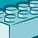# QlikView App Dev

Discussion Board for collaboration related to QlikView App Development.

Announcements
On May 18th at 10AM EDT we will answer your QlikView questions live. REGISTER
cancel
Showing results for
Did you mean:Contributor III

## How to create a synthetic dimension

I need a solution to populating the cycle field (column I).     The cycle formula is based on the units field in (column "D").

Example:

Vendor = 1

Column D = Pounds

Cycle = ( Min (column C) / PoundsDA (column Q) )  *  (calendar day (column K)/ Total Ship Days (column J)

Keep in mind the unit  measure in column D will change and when it does the formula will need to change

Example:

Vendor = 27

Column D = Cube

Cycle = ( Min (column C) / CubeDA (column P) )  *  (calendar day Column K/ Total Ship Days column J)

Columns E- T are expressions that have been moved to variables so for discussion purpose

Column E = V1  (variable 1)

Column F = v2

Column G = V3

Column H = v4

Column I = v5

Column J = v6

Column K = v7

Column L = v8

Column M = v9

Column N = v10

Column 0 = v11

Column P = v12

Column Q = v13

Column R = v14

Column S = v15

Column T = v16

The book "Qlikview Your Business" has some functionality using Synthetic Dimensions and valuelist, match & pick functionality.   Initially I thought this would work but I haven't been able to implement this strategy.     I'm looking for any input or options that would key off the Units fields and allow me to use multiple formulas to populate this field.

troyansky

0 Replies